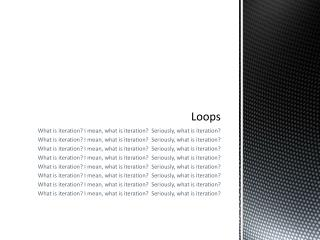DownloadDownload PresentationLoops

# Loops

Download Presentation## Loops

- - - - - - - - - - - - - - - - - - - - - - - - - - - E N D - - - - - - - - - - - - - - - - - - - - - - - - - - -
##### Presentation Transcript

1. Loops What is iteration? I mean, what is iteration? Seriously, what is iteration? What is iteration? I mean, what is iteration? Seriously, what is iteration? What is iteration? I mean, what is iteration? Seriously, what is iteration? What is iteration? I mean, what is iteration? Seriously, what is iteration? What is iteration? I mean, what is iteration? Seriously, what is iteration? What is iteration? I mean, what is iteration? Seriously, what is iteration? What is iteration? I mean, what is iteration? Seriously, what is iteration? What is iteration? I mean, what is iteration? Seriously, what is iteration?

2. Iteration is the process of repeating a set of rules or steps over and over again What is iteration?

3. Loops gives you the ability to repeat a set of code over and over again. • Loops will execute until a condition is met … • If the condition is never met, you have what’s called an endless loop … and that is not good!! • There are three different types of loops • WHILE loop • DO-WHILE loop (really, there is a difference!) • FOR loop Loops

4. The while loop executes the code inside it’s brackets until a condition is met. while (condition) { // if condition is true … do this do that do whatever you want! } //end while loop • So why are they useful? Consider a repetitious situation on the following slide … WHILE loop

5. size(200,200); background(255); // Legs stroke(0); int y = 80; // Vertical location of each line int x = 50; // Initial horizontal location for first line int spacing = 10; // How far apart is each line intlen = 20; // Length of each line // Draw the first leg. line(x,y,x,y + len); // Add spacing so the next leg appears 10 pixels to the right. x = x + spacing; // Continue this process for each leg, repeating it over and over. line(x,y,x,y + len); x = x + spacing; line(x,y,x,y + len); x = x + spacing; line(x,y,x,y + len); x = x + spacing; line(x,y,x,y + len); x = x + spacing; line(x,y,x,y + len); x = x + spacing; line(x,y,x,y + len); x = x + spacing; line(x,y,x,y + len); x = x + spacing; line(x,y,x,y + len); x = x + spacing; line(x,y,x,y + len); x = x + spacing; line(x,y,x,y + len); Why use loops? There must be an easier way!!

6. size(200,200);background(255);  inty = 80; // Vertical location of each lineintx = 50; //Initial horizontal location for first lineintspacing = 10; // How far apart is each lineintlen = 20; // Length of each line // A variable to mark where the legs end.intendLegs = 150; stroke(0);  // Draw each leg inside a while loop. while (x <= endLegs) { line (x,y,x,y + len); x = x + spacing; } Why use loops? Yes, there is an easier way! Same outcome far fewer lines of code!

7. Exit conditions are extremely important! • I don’t want any endless loops … consider the following int x = 0; while (x < 10) { println(x); x = x – 1; } // when will this loop stop? Exit conditions DON’T DO THIS!!

8. Create a program that draws a series of ellipses, each one smaller than the previous. Your end result should look like a target. Extra: create a random number for the colour of the ellipses.See example … trippy man! WHILE loops practice problems

9. The do-while loop is the same as a while loop, but the test comes after the loop, instead of before. • The following is an example … x=0; while (x<10) { x = x + 1; ellipse(x,y,10,10); } //will run 10 times OR x=0; do { x = x + 1; ellipse(x,y,10,10); } while (x<10) //will run 11 times DO WHILE loops … a slight difference

10. Add several balls to yesterday’s program. • Add a user controlled ball to the screen that tries to cross the screen, a la frogger. More while loops practice

11. Much like While loops, FOR loops are a block of code that repeats. • The difference is that a FOR loop executes a given number of time. Example:void setup () { size(500,500); frameRate(4); } void draw() { fill(0); for (int x=100; x > 0; x=x-5){ fill(random(0,255),random(0,255),random(0,255)); ellipse(100,100,x,x); } } • Practice: combine this with your maze. Make your character flash colour like in this example. • Practice: make Zoog’s eyes change colour hypnotic style FOR loops